# Automate PowerPoint Production Using R

Author

Joseph Noonan

Published

February 17, 2021

One of the constants in my professional career is making graphs to put into PowerPoint presentations so that findings can be presented to other teams, partners, or clients. The difficultly with making PowerPoint presentations it is done manually and takes a ton of time. While R Markdown can allow streamlined workflows, it doesn’t always work in collaborative settings where PowerPoint is king. This is where the `officer` package comes to the rescue.

The goal for this post is to show you how to use for loops to automate graph production in `ggplot2` and to take those graphs and put them into a PowerPoint deck with the `officer` package. This tutorial will be best suited to intermediate R users. You should be fairly comfortable with `ggplot2` and the basics of loops or you might get lost when we start automating some of the processes.

This process can be broken down into 4 steps:

1. Get the data
2. Make a loop that makes the graphs
3. Prepare the PowerPoint
4. Make a loop that adds the graphs to the PowerPoint

The final product will be a single PowerPoint with a slide for each country showing the GDP annual % growth from 2009-2019.

At the end of the post, I’ll go through briefly another way of tackling this problem using functional programming instead of for loops.

All of the code used here is also avaiable on GitHub, including the PowerPoint materials

### 1. Get the data

To start with we are going to need to get some example data. Since I’ve always worked in the political science world, we are going to look at data from the World Bank. The `wbstats` package makes grabbing data from the World Bank’s database very easy.

``````# Create df using wbstats
# create new variable so that that gdp_pct_growth is formatted as %
library(tidyverse)
library(wbstats)
gdp_pct_growth_df <- wbstats::wb_data(
country = "countries_only",
indicator = "NY.GDP.PCAP.KD.ZG",
start_date = 2009,
end_date = 2019,
return_wide = FALSE) %>%
mutate(gdp_pct_growth = value / 100)``````

Here I am grabbing the GDP annual % growth rate, only for countries (excluding regional classifications), between 2009 and 2019. You can change what indicators you want to pull by using the `indicator` argument. To find the indicator codes you can either use the function `wb_search` or just go to the World Bank’s online database and find it there. Since there are so many different indicators, I actually prefer using the web interface.

The result is a long dataset with a row for each indicator–country–year. In this case we are only working with one indicator but I still prefer working with long data since it works better with `ggplot2` when graphing multiple indicators. Below, you can see how the dataset looks based on a random sample of rows. I have only included the most relevant variables.

``````gdp_pct_growth_df %>%
select(indicator, country, iso3c, date, gdp_pct_growth) %>%
sample_n(5)``````
indicator country iso3c date gdp_pct_growth
GDP per capita growth (annual %) Curacao CUW 2019 -0.0220876
GDP per capita growth (annual %) Mexico MEX 2017 0.0101582
GDP per capita growth (annual %) Japan JPN 2009 -0.0568145
GDP per capita growth (annual %) Tanzania TZA 2019 0.0265632
GDP per capita growth (annual %) Gibraltar GIB 2013 NA

### 2. Make a loop that makes the graphs

When I write loops to mass produce graphs, I follow a simple two step process: first, write the code to make the graphs, then figure out what actually need to change to make a the same graph but with different parameters This may seem simple, but I think for beginners it is important to break it down so you don’t get lost in trying to both learn how to do a task and automate it at the same time.

So, I will start with making a graph for a single country using the code below.

``````# Create a df with only data for France
df <- filter(gdp_pct_growth_df, country == "France")

# Create a simple line chart showing trends from 2009-2019
# geom_hline marks 0% growth for reading ease
# axis.title.x= element_blank() removes the x-axis (Year) title label
# axis.text.x = angle = 90 rotates the axis x text

ggplot(df, aes(x = date, y = gdp_pct_growth)) +
geom_line(size = 1) +
geom_point(size = 3) +
geom_hline(yintercept = 0, linetype = "dashed", color = "red", size = 1) +
scale_x_continuous("Year", breaks = seq(2009, 2019, 1), limits = c(2009, 2019)) +
scale_y_continuous("GDP per capita growth (annual %)", labels = scales::percent) +
labs(
title = "GDP per capita growth (annual %)",
subtitle = "France, 2009-2019",
caption = "Source: World Bank Open Data"
) +
theme_minimal() +
theme(
axis.title.x = element_blank(),
axis.text.x = element_text(angle = 90)
)``````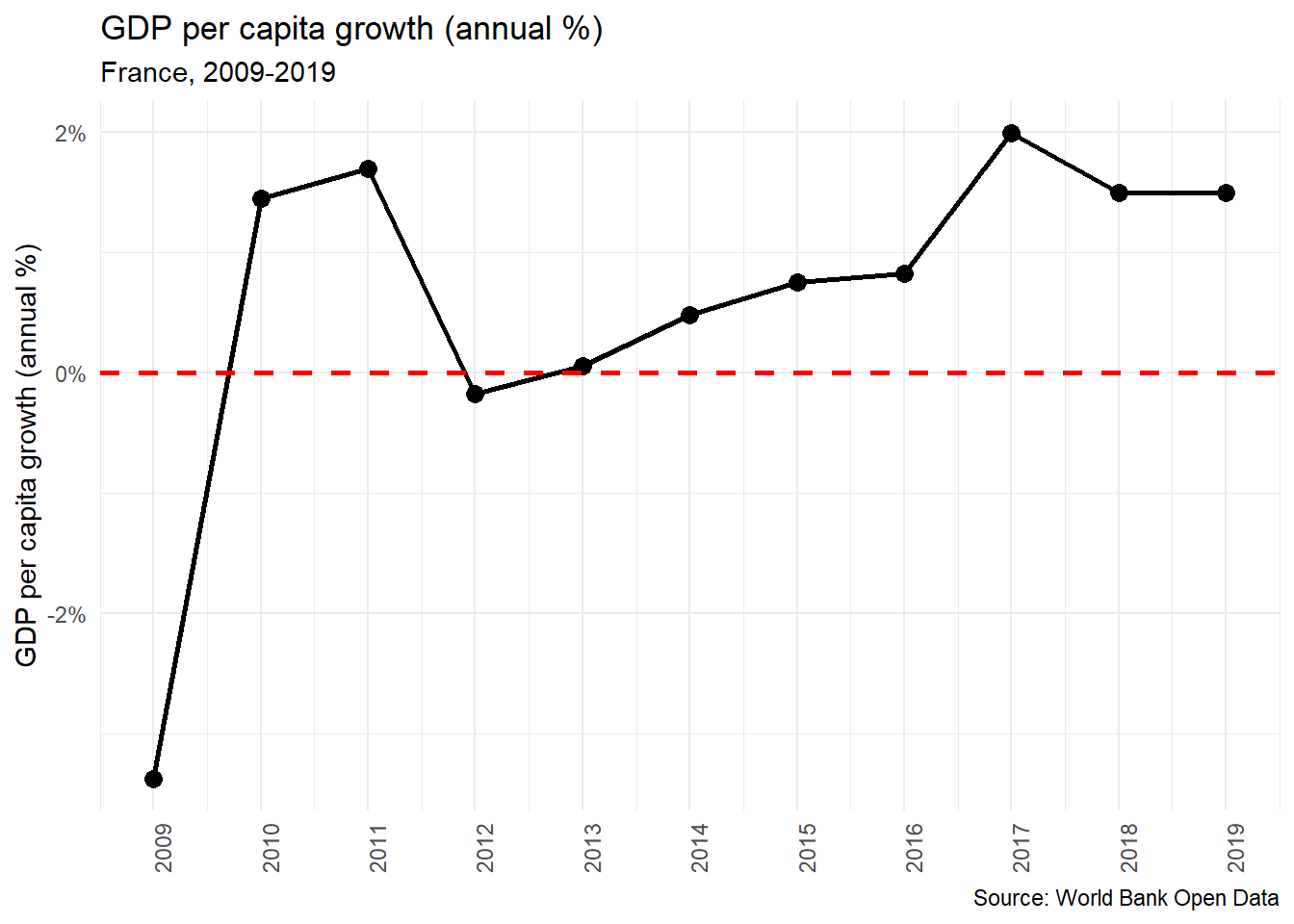Now we have a very simple line chart of the GDP per captia growth in France from 2009-2019. The next step is making a chart like this for every country in the dataset, save that graph, and make a list of the file paths for all of these charts. This is where we are going to use a for loop. What for loops allow us to do is to repeat a task over and over again with different parameters. The first step in this process is to we need to first identify what parameters we will need to change for each graph. In this example there are three points where we have fill in, creating the dataset for the chart, the subtitle which mentions the country’s name, and the file name when we save our plot.

``````# Make a list of all unique countries
country_list <- unique(gdp_pct_growth_df\$country)``````

In this first step we are creating a list of countries which we will use to tell R what to iterate through. Here we are using the `unique()` function which will give us all of the unique values for the variable `country` in our dataset, that is to say, all unique country names.

``````# Create a blank file path list to append to
file_path_list <- list()
``````

Next, we are going to make a blank list. We will use this in our loop to create a list of file paths for all of the graphs we create. We will use this later when we build our PowerPoint decks with the `officer` package.

The next code chunk is the full for loop for creating and saving the graphs.

``````# For loop that produces graphs for every country
for (i in seq_along(country_list)) {

# Step 1
# For each iteration, create a df for only that country

df <- filter(gdp_pct_growth_df, country == country_list[i])

# Step 2
# For each iteration, create a plot
# Use paste0(country_list[i]), to change labels when needed

plot <- ggplot(df, aes(x = date, y = gdp_pct_growth)) +
geom_line(size = 1) +
geom_point(size = 3) +
geom_hline(yintercept = 0, linetype = "dashed", color = "red", size = 1) +
scale_x_continuous("Year", breaks = seq(2009, 2019, 1), limits = c(2009, 2019)) +
scale_y_continuous("GDP per capita growth (annual %)", labels = scales::percent) +
labs(
title = "GDP per capita growth (annual %)",
subtitle = paste0(country_list[i], ", ", "2009-2019"),
caption = "Source: World Bank Open Data"
) +
theme_minimal() +
theme(
axis.title.x = element_blank(),
axis.text.x = element_text(angle = 90)
)

# Step 3
# Store file path
# str_replace_all replaces countries names so that it is alpha numeric to avoid invalid file path issues.

plot_file_path <- paste0(getwd(), "/graphs/", str_replace_all(country_list[i], "[^[:alnum:]]", "_"), ".png")

# Append file path to list for each iteration
file_path_list <- append(file_path_list, list(plot_file_path))

# Step 4
# Save the graph
ggsave(filename = plot_file_path, plot = plot, width = 7, height = 5, units = "in", scale = 1, dpi = 300)
}
``````

Here is how the loop works:

1. It starts by creating a data frame for the iteration. To do this we use the `filter` function to filter the dataset based on the country in `country_list[i]`

2. Next it creates the plot. The code here is almost identical to the the we used to make the the graph for France. The only difference is that we need to change the subtitle so that it changes based on the country the loop is iterating through. To do this we use the `paste0` function, this allows us to concatenate strings pragmatically, so that we have both the country name and the years that it covers in the subtitle.

3. The next step is to create a file path and store it as object. Here we are using the `paste0()` function again. We first grab the working directory with `getwd()`, add in the subfolder ‘graphs’ and then lastly, name the image file. Here we are using the `str_replace_all()` function from the `stringr` package to remove all non-alphanumeric characters from the country names. Saving files with apostrophes or periods can often result in errors, so it is best to just avoid them completely. Once we have created our file path, we append it to the master lists of file paths that include all iterations in the loop.

4. Lastly, we save the graphs. Take note of the width and the height, as that is something we will need to be aware of when we prepare our PowerPoint.

The result is a graph for every country in our dataset saved to your hard drive and a list of file paths for each of those graphs (`file_path_list`).

### 3. Prepare the PowerPoint

When manipulating PowerPoints using the `officer` package, we need to create a ‘template’ PowerPoint deck, where we can specify the layout that we want our slides to have. In PowerPoint you do this through the ‘slide master’ view. If you don’t know how layouts in PowerPoint work, Microsoft provides a good overview of how they work. Since the titles of the graphs are in the image themselves, we can just make a new layout type with a content box that has the same dimensions as the graphs that we saved (7 inches by 5 inches). If the dimensions don’t match you’ll end up with some weird scaling and warping issues.

Here is what the template PowerPoint we will be working with looks like in the slide master view.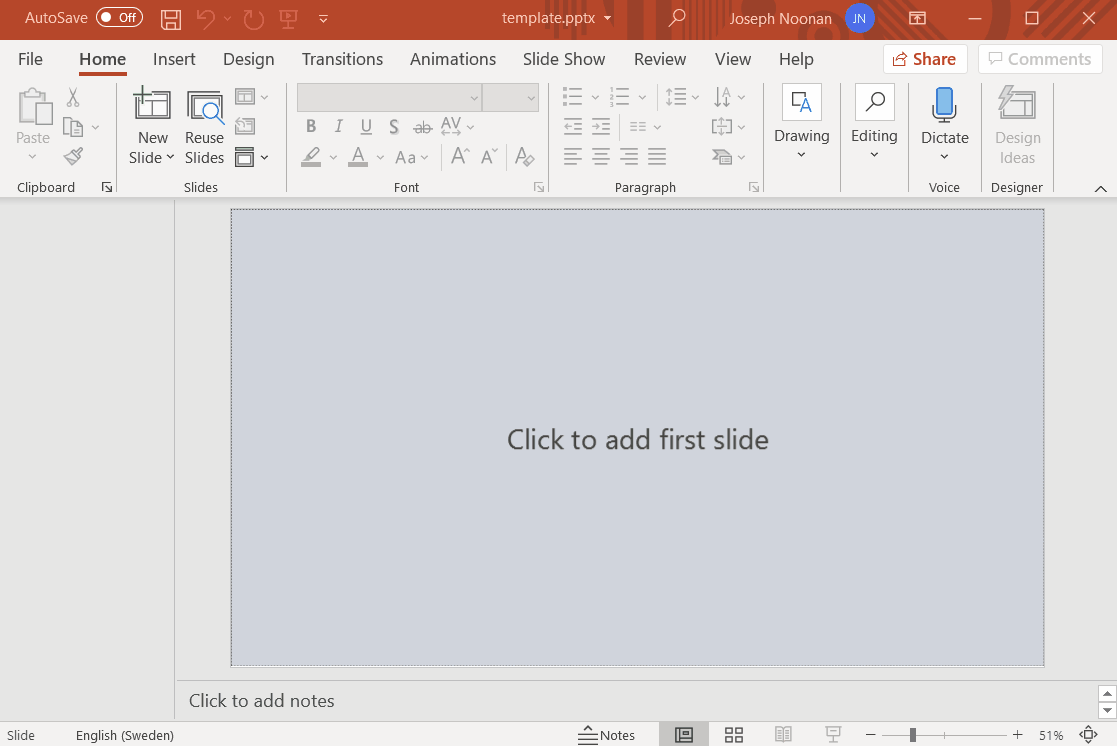Once we have created PowerPoint template deck we can use `read_pptx()` to read in our PowerPoint into R.

``````library(officer)

Next, we can use the `layout_summary()` function to take a look at the different types of slide layouts we can use.

``````# Look at summary of slide layouts
officer::layout_summary(template_pptx)
``````
layout master
Title Slide Retrospect
Two Content Retrospect
Graph Retrospect

Here we have three different layouts, all from the same slide master “Retrsospect”. The custom slide that I have made is the layout “Graph”, while the other two are default layouts. We can take a closer look at the different elements with the “Graph” layout using `layout_properties()`.

``````# Look at layout properties
officer::layout_properties(x = template_pptx, layout = "Graph")``````
master_name name type id ph_label
3 Retrospect Graph body 10 Content Placeholder 2
7 Retrospect Graph body 5 Rectangle 4
8 Retrospect Graph body 6 Rectangle 5

This table shows us the different elements in the “Graph” slide layout. The most important element is “Content Placeholder 2” which is where the graph will go in the slide. The two rectangle elements are just aesthetic elements in the footer of the slides.

### 4. Make a loop that adds the graphs to the PowerPoint

Now that we have the information about the layout of the PowerPoint template we can build our loop. We are going to be using four different functions from the `officer` package:

• `add_slide()` - This function is fairly self explanatory, it adds a slide to a pptx object. In this case we have read in our pptx object as `template_pptx`.
• `ph_with()` - This function adds a new shape or object into the existing slide. The two arguments will be using are `value` and `location`
• `external_img()` - This function reads in a specified external image so that it can be embedded into a PowerPoint slide.
• `ph_location_label()`. - This function is used in conjunction with ‘ph_with()’ to specify where an object should be added into the PowerPoint slide.

Something that is a bit confusing (for me at least) is how `add_slide()` adds a slide the to the specified PowerPoint and then overwrites the original PowerPoint object. I think this is best illustrated with an example.

I’ll start by looking at a summary of the blank PowerPoint `template_pptx`, using `pptx_summary()`.

``officer::pptx_summary(template_pptx)``
``NULL``

Since there is are no slides, we get nothing returned. Now I’ll add one slide.

``````officer::add_slide(template_pptx,
layout = "Title Slide",
master = "Retrospect") %>%
officer::ph_with(
value = "Test Title 1",
location = ph_location_label(ph_label = "Title 1")
)

officer::pptx_summary(template_pptx)``````
text id content_type slide_id
Test Title 1 2 paragraph 1

Now we have one slide containing only a title. If we were to rerun the code that we just ran, we would add the same slide again. It wouldn’t overwrite anything we just would have two of the same slides.

``````officer::add_slide(template_pptx,
layout = "Title Slide",
master = "Retrospect") %>%
officer::ph_with(
value = "Test Title 1",
location = ph_location_label(ph_label = "Title 1")
)

officer::pptx_summary(template_pptx)``````
text id content_type slide_id
Test Title 1 2 paragraph 1
Test Title 1 2 paragraph 2

This is important to know both when for writing loops, but also when using the `add_slide()` function in general. You have to take extra care that your script is written in the order that you want the slides to appear, and when you are experimenting you need to be sure to clear out the pptx object by reading in the blank one so you don’t end up having a ton of ‘work in progress’ slides in your deck.

Now that we know how `add_slide()` works, it is time to write a loop. Before we used `country_list` to iterate through our loop but this time we are going to use the `file_path_list` that we created earlier. This is because the one parameter that we are changing is no longer country names, but rather where the image files are on our computer.

``````# Copy blank pptx to gdp_growth_pptx (which will be our final pptx)
gdp_growth_pptx <- template_pptx

for (i in seq(file_path_list)) {
plot_png <- external_img(file_path_list[[i]])

layout = "Graph",
master = "Retrospect") %>%
ph_with(
value = plot_png,
location = ph_location_label(ph_label = "Content Placeholder 2")
)
}``````

We start first by copying the `template_pptx` to a new object called `gdp_growth_pptx`. Because `add_slide()` is adding slides to the PowerPoint object, it is good to have a template object that you never change. The loop itself works in two steps. First read in saved ggplot2 image using `external_img()` then add it to the slides using `add_slide()` to specify the PowerPoint object and the layout, and `ph_with()` to specify what should be embedded and where it should be embedded in the slide.

The final product is single PowerPoint with a 217 slides, one for each country showing the GDP annual % growth from 2009-2019. To export this PowerPoint object you use the `print()` function.

``````# Export
print(gdp_growth_pptx, target = "gdp_per_capita_%_growth_slide_deck.pptx") ``````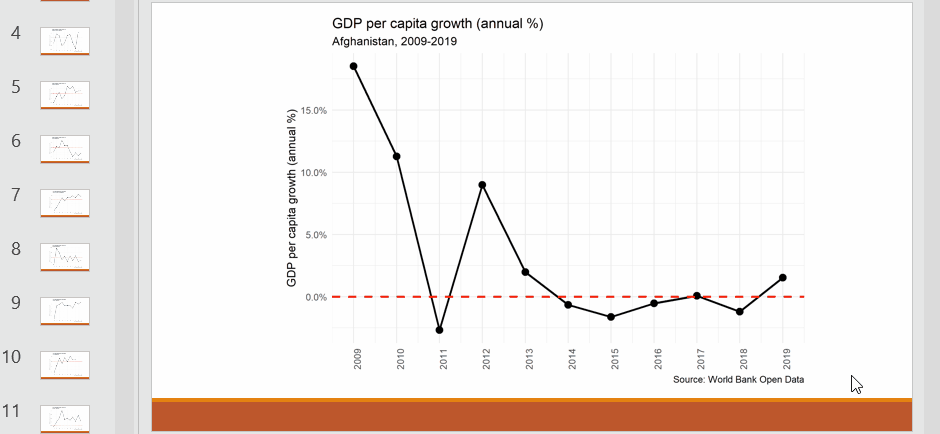This example is probably the simplest automation of PowerPoint reports you could make, only one paremeter changes when creating the graphs using `ggplot2` and we are assuming that ther order doesn’t matter. However, this is a good starting point, reports with more complexity can be made using the techniques outlined in this post.

### Bonus. Avoiding (some) loops by using the map function

For loops in R are often discouraged because they can be overly verbose and result in a ton of copying and pasting where small parameters are tweaked. An alternative approach to for loops is to use functionals like the `map()` functions from the `purrr` package. How these functions work is neatly summed up in the excellent book R for Data Science:

Each function takes a vector as input, applies a function to each piece, and then returns a new vector that’s the same length (and has the same names) as the input.

In our case we will create a custom function with the same parameters as the for loop we made in Step 2, this will be called `country_graph()`. Then we will use use the `map()` function, input our ‘country_list’ vector, and use `country_graph()`. The end result will give us a list of `ggplot2` graphs, one for each country in our dataset.

Rewriting our loop as a function, isn’t super difficult. In practice, this means rewriting our code so that instead of using `country_list[i]` for the parameters that we need to change between iterations, we use `x`. This function has also been reworked so that we aren’t saving the files to the hard drive in this function, but rather just creating a ‘ggplot2’ plot.

``````# Create a function to make the graph

country_graph <- function(x) {

# X argument is country name

df <- filter(gdp_pct_growth_df, country == x)

ggplot(df, aes(x = date, y = gdp_pct_growth)) +
geom_line(size = 1) +
geom_point(size = 3) +
geom_hline(yintercept = 0, linetype = "dashed", color = "red", size = 1) +
scale_x_continuous("Year", breaks = seq(2009, 2019, 1), limits = c(2009, 2019)) +
scale_y_continuous("GDP per capita growth (annual %)", labels = scales::percent) +
labs(
title = "GDP per capita growth (annual %)",
subtitle = paste0(x, ", ", "2009-2019"),
caption = "Source: World Bank Open Data"
) +
theme_minimal() +
theme(
axis.title.x = element_blank(),
axis.text.x = element_text(angle = 90)
)
}``````

We can use this function on it’s own if we want to make graphs for specific countries. This can be a practical alternative if you don’t need to create graphs for all of the different groups in a given dataset.

``````# Create a graph for Zimbabwe
country_graph("Zimbabwe")``````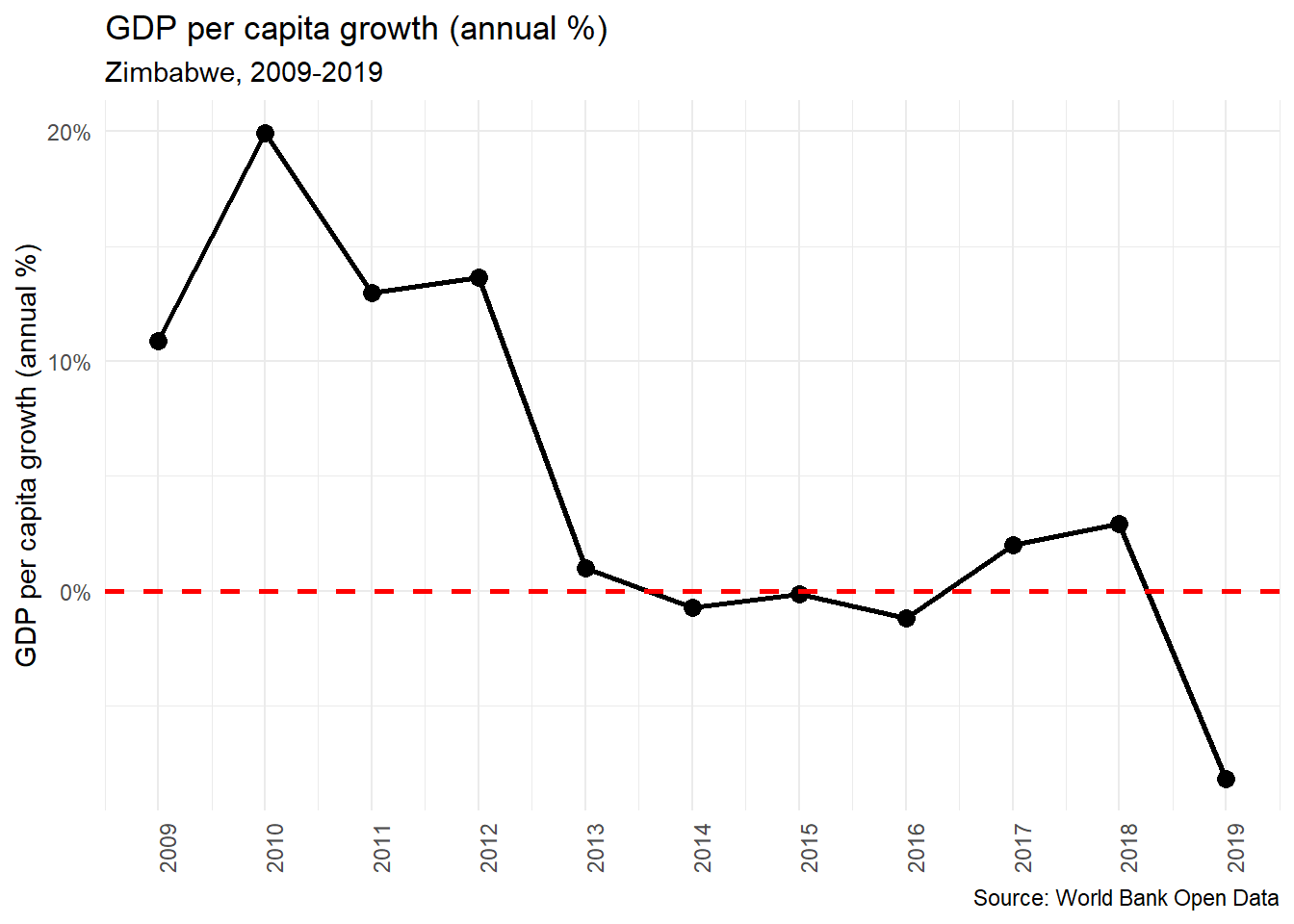In the next step, we use `map()` to apply `country_graph()` to `country_list` and save those results as `country_graph_list` (please excuse my very creative naming conventions).

``````# Use map to make graphs
# set_names() sets the names of a list based on their values
country_graphs_list <-  set_names(country_list) %>%
purrr::map(country_graph)``````

Now we have a a list of `ggplot2` graphs. This means we can call the plots for each country by calling elements in the list.

``country_graphs_list\$Uganda``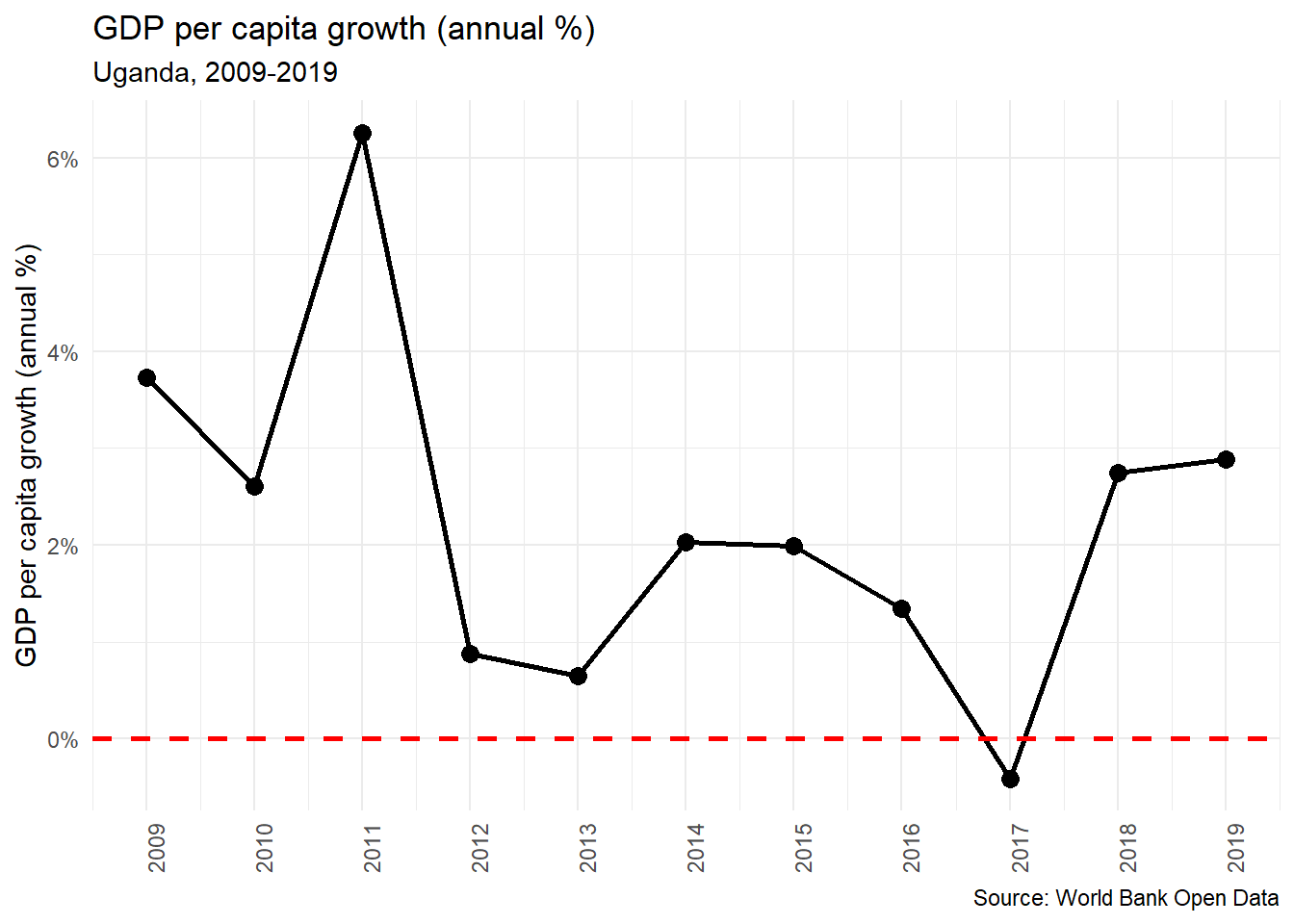To take this list and put all of the `gglot2` graphs into a PowerPoint we use a similar loop as we used before, but this time we are a looping over the `country_graph_list` and instead of adding in external images, we are directly calling the `ggplot2` object in the `value` parameter of `ph_with()`.

``````# Copy blank pptx to gdp_growth_pptx (which will be our final pptx)
gdp_growth_pptx <- template_pptx

for (i in seq(country_graphs_list)) {
While this approach is more streamlined and seemingly simpler, the reason I prefer using external images is that the proportions and the `ggplot2` are easier to control. When using this technique the titles and subtitles are much smaller in the PowerPoint and I am not sure the best way to fix that. But, like all things in R, I am sure there is just a solution I haven’t figured out yet.